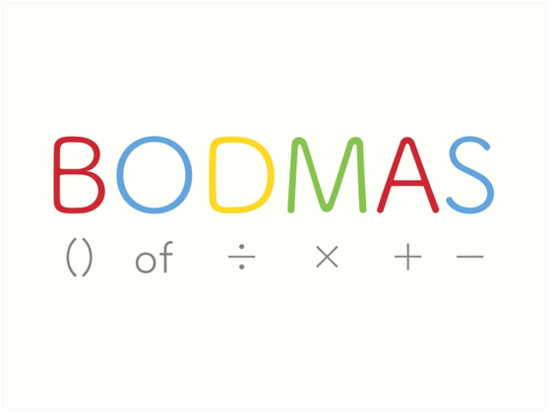# BODMAS Rule – Definition and FormulaThere are various operations in Mathematics with the help of which we solve different kinds of equations. When we solve equations, we need to use the operations in order so that there is a similarity in answers and students do not get confused when it comes to solving equations. Hence a set of rules is formed called the order of operations.

The order of operations helps us solve an equation using the proper order of operations in order to avoid confusion and maintain familiarity in answers. There are different orders of operations such as BODMAS, PEMDAS, etc.

# BODMAS Rule – Definition and Formula

This order of operations is important because it helps students understand and solve complex equations with ease. Without BODMAS, there can be a lot of confusion among students, and they might end up getting the wrong answers.

BODMAS is introduced early in a student’s mathematical journey as it helps them solve equations with ease. Also, having a solid foundation of BODMAS helps students solve complex equations in the future.

BODMAS helps students increase their speed and is also beneficial as it increases the accuracy of students, thus helping them perform better. In this blog, we are going to explore the concept of BODMAS in an interesting and engaging way.

## What is BODMAS?

BODMAS is a set of rules that helps students solve different types of equations. BODMAS stands for Brackets of or order, Division, Multiplication, Addition, and Subtraction. The full form itself is an indication of the order of the operations that need to be performed in an equation. BODMAS is one of the most exciting concepts in Mathematics, and mastering BODMAS gives students a lifelong advantage over others. BODMAS is known by different names, such as PEMDAS in the US. Both BODMAS and PEMDAS do the same job except for the names.

BODMAS becomes simple and easy with Cuemath. Cuemath is an online learning platform that engages students most interestingly. Cuemath uses modern learning tools like visuals, simulations, puzzles, and games that make learning super fun and interesting. Not just that, Cuemath helps students understand the why behind the what of the concepts that make learning interesting. You can master the concept of BODMAS using worksheets by Cuemath. These worksheets are elaborate, interactive, and super fun to solve. You can visit the Curmath website from where you can download these worksheets for free.

## Rules of BODMAS

BODMAS lays a set of rules which needs to be followed in order to solve equations correctly. This set of rules tells us which operation needs to be performed first. BODMAS rules follow the order in which they are written, such as B stands for Brackets of or order, Division, Multiplication, Addition, and Subtraction. Solving equations with the help of BODMAS can help them with accuracy.

• Step 1 Brackets of In the first step, all the brackets, and braces are simplified. This also includes the set of symbols which are placed inside the bracket. Hence we need to solve the brackets first in order to solve the equation.
• Step 2 Orders: In the second step, the numbers that include powers and roots, we need to simplify all the numbers involving power and roots. Once we solve the power and roots, we now move on to multiplication and division.
• Step 3 Multiplication and Division: Now, all the terms, including multiplication and division, need to be solved.
• Step 4 Addition and Subtraction: In the last step, we need to solve two operations, i.e., addition and subtraction.

I hope you had fun exploring BODMAS and its concepts. If you have any doubts, you can comment down below. Happy Learning!